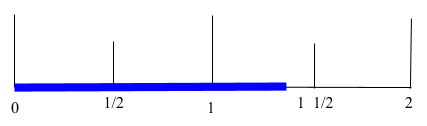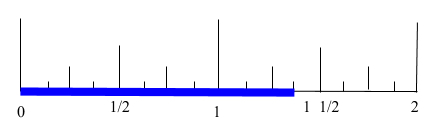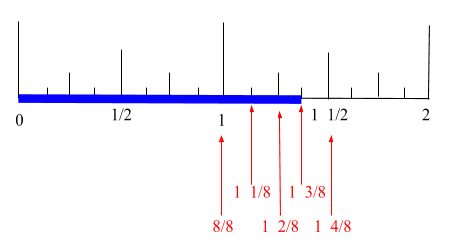Support Union Test Prep

Support Now

# Page 1-Measurement Study Guide for the Math Basics

## How to Prepare for the Measurement Questions on a Math Test

### General Information

Measuring is just using numbers to describe something—a size, a weight, a volume, etc. It’s the different units that can trip you up. Something that measures 7 meters is huge compared to something that measures 7 millimeters and you need to know the difference.

### Measuring Length and Distance

If you want to know how tall your friend is, how long a bowling alley is, or how far away the closest gas station is, you’ll need to measure length: the dimension for distance.

Most of the world (with the exception of U.S.A., Burma, and Liberia) use the metric system. It’s an easy system to use because it’s based on the number 10, like our number (decimal) system. Here are some common conversions for the metric system:

$10\; \text{millimeters} = 1\; \text{centimeter}$ $100 \; \text{centimeters} = 1\; \text{meter}$ $1,000 \; \text{meters} = 1\; \text{kilometer}$

In the United States, we use United States Customary Units. Here are some common conversions for the USC system:

$1 \; \text{foot}= 12\; \text{inches}$ $3 \; \text{feet} = 1\; \text{yard}$ $5,280 \; \text{feet} = 1 \; \text{mile}$ $1,760 \; \text{yards} = 1 \; \text{mile}$

Occasionally, you’ll have to navigate between the two. Use this chart for common conversions between metric and USC units:

$1 \; \text{inch} = 2.54 \; \text{centimeters}$ $1 \; \text{mile} = 1.61 \; \text{kilometers}$ $1 \; \text{foot} = 30.48 \; \text{centimeters}$

Use this table for abbreviations:

$\begin{array}{|c|c|} \hline \textbf{Units} & \textbf{Abbreviation} \\ \hline \text{millimeters} & \text{mm} \\ \hline \text{centimeters} & \text{cm} \\ \hline \text{meters} & \text{m} \\ \hline \text{kilometers} & \text{km} \\ \hline \text{inches} & \text{in} \\ \hline \text{yards} & \text{yd} \\ \hline \text{miles} & \text{mi} \\ \hline \end{array}$

You’ll need to know how to use a ruler or a tape measure when measuring smaller distances. Here’s a walkthrough on how to use a standard inch ruler. Find the length of this blue line segment.You can see that the length is more than 1 inch but less than 2 inches. So, take a look at the partitions below that are almost as tall as the inch markings. Each of these markings stands for a half inch.Notice the length is between $$1$$ and $$1 \frac{1}{2}$$ inches. To get even more precise, look at the next tallest markings which stand for quarter inches.At this point, most rulers and tape measures won’t label these distances. But each time the line markings become shorter, the distances halve (tallest lines are $$1$$, second tallest are $$\frac{1}{2}$$, third tallest are $$\frac{1}{4}$$, etc.). Look at this image to see the lengths.So you can see that the length is between $$1 \frac{1}{4}$$ and $$1 \frac{1}{2}$$. Let’s get more precise. The next tallest partition is eighths ($$\frac{1}{8}$$’s).Now, remember the length was somewhere between $$1 \frac{1}{4}$$ and $$1 \frac{1}{2}$$. If we write the partitions (below) with the same denominator (as we did for quarter inches), we can see precisely which number it is.So the blue line is $$1 \frac{3}{8}$$ inches long.

Note: Some tape measures or rulers go all the way to sixteenths ($$\frac{1}{16}$$’s), but you get the jist by now.

### Measuring Liquids and Capacity

If you’ve ever followed a recipe, you’re familiar with using units to measure liquids and capacity. This section will contain all common conversions you’ll need. To find out how to convert the units, refer to the Dimensional Analysis section at the end of the study guide.

As usual, the United States and most of the rest of the world use different systems. Here are some common U.S. Standard conversions for liquids.

$1 \; \text{tablespoon}=3 \; \text{teaspoons}$ $1\; \text{fluid ounce} = 2 \; \text{tablespoons}$ $1\; \text{cup} = 8 \; \text{fluid ounces}$ $1 \; \text{pint} = 2 \; \text{cups}$ $1 \; \text{quart}=2\; \text{pints}$ $1 \; \text{gallon} = 4\; \text{quarts}$

Use this chart for abbreviations.

$\begin{array}{|c|c|} \hline \textbf{Units} & \textbf{Abbreviation} \\ \hline \text{teaspoon} & \text{tsp} \\ \hline \text{tablespoon} & \text{Tbsp} \\ \hline \text{fluid ounce} & \text{fl oz} \\ \hline \text{cup} & \text{cup} \\ \hline \text{pint} & \text{pt} \\ \hline \text{quart} & \text{qt} \\ \hline \text{gallon} & \text{gal} \\ \hline \end{array}$

Now, for the metric conversions (much easier):

$1\; \text{centiliter} = 10 \; \text{milliliters}$ $1 \; \text{liter} = 1000 \; \text{milliliters}$

Easy, right?! Here are the abbreviations:

$\begin{array}{|c|c|} \hline \textbf{Units} & \textbf{Abbreviation} \\ \hline \text{centiliter} & \text{cl} \\ \hline \text{milliliter} & \text{ml} \\ \hline \text{liter} & \text{l or L} \\ \hline \end{array}$

To get from U.S. standard to metric and back, the following might be helpful.

$1\; \text{fl oz} = 30 \; \text{ml}$ $1 \; \text{pt} \approx 500 \; \text{ml}$ $1 \; \text{qt} \approx 1 \; \text{L}$

## All Study Guides for the Math Basics are now available as downloadable PDFs

View other purchase options